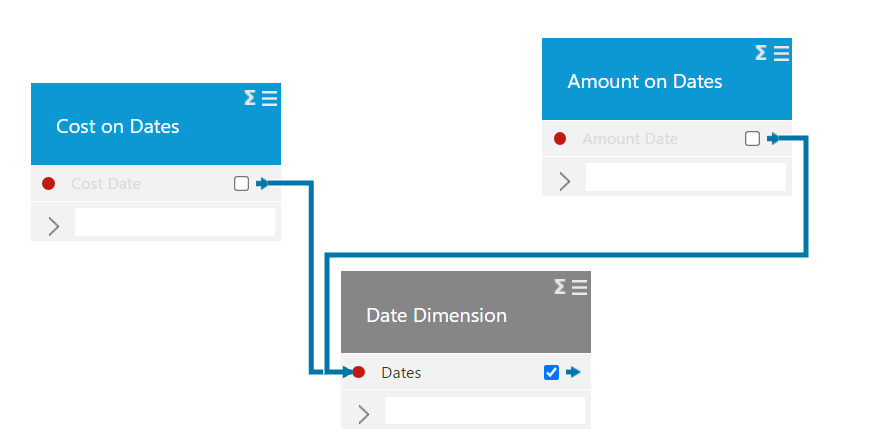# Relations between Fact tables and Dimension tables - wrong way and right way

When you build data models, there are some conventions you need to follow to be sure that the data model will always return correct results.

You need to understand concepts like:

• FACT tables and DIMENSION tables
• Many-to-Many and Many-to-One relationships

Below is a simple explanation of these concepts.

Let's look at a very simple scenario where you have 2 data sources - both containing numbers that are needed for analysis (which makes the into FACT tables):

Data source 1 (Amount)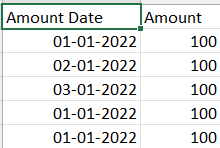Notice in this data source that there are 3 entries for the 3rd of January. In other words - the Amount Date is not unique - there can be more records on the same day.

Date source 2 (Cost)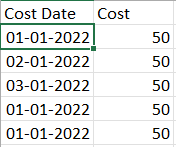Notice again that this is pretty much the same - more entries on one day - the date is not unique.

## The Wrong Way!

Now the wrong way to connect these data sources is like this (fact-to-fact):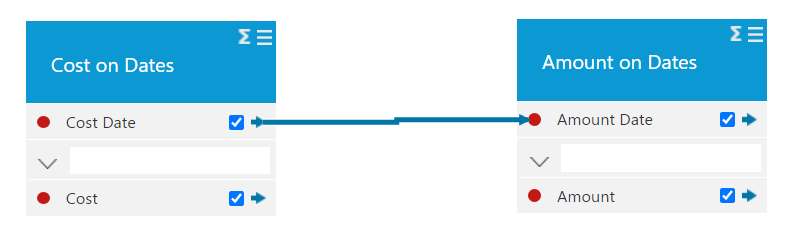Now we have dragged a connection between to non-unique columns both which is called a many-to-many relation.

TARGIT Data Discovery does not support setting up many-to-many relations, so we are unable to guarantee that this model will give you the right result.

Furthermore if this (instead of just 3 dates) we're data sources containing thousands or even millions of entries, this results in extreme use of RAM when you make these relations.

Unfortunately making relations directly between fact tables can sometimes give the right result even though it is basically WRONG!

## The Right Way!

When you want to work with numbers from more than 1 fact table - you need to introduce a DIMENSION table.

In this case it could just look like this: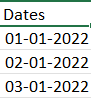The characteristics of a Dimension table is - that it DOESN'T contain numbers that should be used for measures - and that the column you use for relation to fact tables is UNIQUE.

The very small table above meets both criteria's and now we are ready for the right way: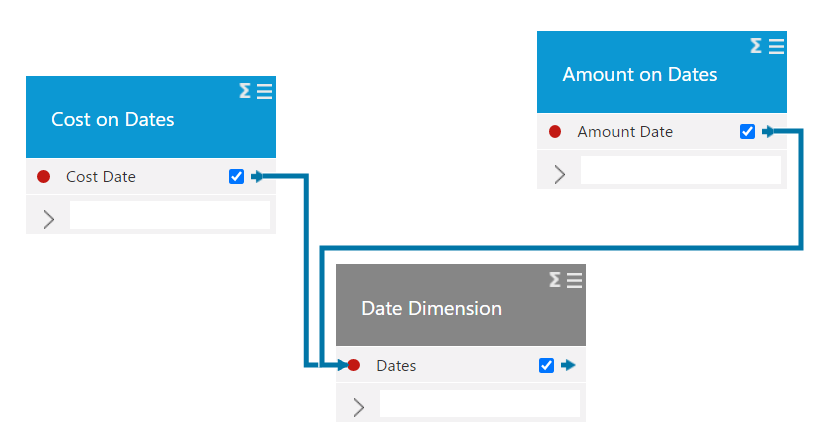Notice that the relations are dragged from MANY-TO-ONE -from the fact table column to the dimension table column!

One final touch would be to hide both Cost Date and Amount Date so you don't accidentally try to use those as dimensions, which will again potentially give you WRONG results.

Here they are unticked and the cube can be saved and is ready for use: